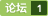### 关于python利用动态变量实时绘图的问题 [问题点数：50分]python中plot实现即时数据动态显示方法

python学习：使用Matplotlib画实时动态折线图

<em>python</em>学习：使用Matplotlib画<em>实时</em>的<em>动态</em>折线图 有时，为了方便看数据的变化情况，需要画一个<em>动态</em>图来看整体的变化情况。主要就是用Matplotlib库。 参考博客链接：https://blog.csdn.net/zhanghao3389/article/details/82685072 https://blog.csdn.net...

python绘制动态曲线

python动态生成波形曲线

效果是这个样子的： 用到的模块: * matplotlib.pyplot * matplotlib.animation.FuncAnimation * numpy 三个圆的半径分别为：5， 2， 1。 三个点旋转的角频率分别为：1， 3， 5。 生成固定的圆——基础圆 xdata = np.linspace(0, 2 * np.pi, 50) fig, ax = pl...

python绘制动态模拟图

pyqtgraph逐点画波形图例子

python获取新浪A股实时股票数据并实时画图

python 画3维轨迹图并进行比较

Python中matplotlib实时画图

github博客传送门 博客园传送门 <em>实时</em>画图 import matplotlib.pyplot as plt ax = [] # 定义一个 x 轴的空列表用来接收<em>动态</em>的数据 ay = [] # 定义一个 y 轴的空列表用来接收<em>动态</em>的数据 plt.ion() # 开启一个画图的...

# -*- coding: UTF-8 -*- import wave import numpy as np import matplotlib.pyplot as plt # 打开wav文件 ，open返回一个的是一个Wave_read类的实例，通过调用它的方法读取WAV文件的格式和数据。 f = wave.open(r&quot;D:\project\REC001.wav&quot;,&quot;rb&quot;) # 读取格式...

python+opencv如何画出实时视频小车轨迹

python利用动态变量实时绘图问题-读取数据库数据

importpymysql importthreading importtime importnumpyasnp importmatplotlib.pyplotasplt frommatplotlibimportanimation frommatplotlib.patchesimportCircle fig,ax=plt.subplots() r=0 #pyth...

Python学习笔记#5：matplot动态图形显示

Python使用matplotlib画动态

python3使用matplotlib.pyplot绘制动态

<em>实时</em>读取显示波形和频谱 环境： Ubuntu16.04 <em>python</em>2.12 matplotlib 源码： https://github.com/lightjiang/AudioProcess 效果图 发 呜呜～ 音， 低频部分明显上升 傅里叶变换直接调用的numpy.fft工具，大概就能看个分布趋势吧 matplot动图更新频率因为帧读取长度选

python实现从串口读取数据并绘制动态曲线，同时保存数据，曲线绘制可实现暂停

python绘图动起来

Visdom画动态折线图

from visdom import Visdom import numpy as np import time vis=Visdom(env="loss") x,y=0,0 win=vis.line(np.array([y]),np.array([x])) for i in range(100): x=np.array([i]) time.sleep(0.1) y=...

PYQT5+matplotlib实时绘制三维动态曲线图

<em>问题</em>分析 自动轨迹绘制 -需求： 根据脚本来绘制图形？ -不是写代码而数据绘制轨迹 -数据脚本是自动化最重要的第一步

【初学python】实例十：自动轨迹绘制

Python-Matplotlib 动态图表绘制来达到实时监控

python实现可暂停的动态曲线绘制，横纵坐标从上一个点到当前点，同时保存数据到文件中

<em>python</em>实现可暂停的<em>动态</em>曲线绘制，横纵坐标从上一个点到当前点，同时保存数据到文件中

Python可视化-折线图

Plotly Dash 画多个子图

import plotly.plotly as py import plotly.graph_objs as go trace1 = go.Scatter( x=[1, 2, 3], y=[4, 5, 6] ) trace2 = go.Scatter( x=[20, 30, 40], y=[50, 60, 70], xaxis='x2', yax...

python – 如何使用matplotlib在while循环中实时绘图

Qt绘制实时曲线图形简单例程 - Qt实时绘图

本来是想写一篇上位机通过串口接收数据并进行简单<em>实时</em><em>动态</em>画图的，但是网上关于Qt串口接收数据的博客已经非常多了，且也会导致内容太多，显得复杂，如果需要串口收发数据例程，网上随便百度就能收到了，也可以下载我写的北斗/GPS双模定位上位机，这里主要介绍一下收到数据后的画图实现。        使用Qt绘制图形，首先需要了解Qt的窗口与视口的概念，理解Qt的坐标变换，然后学会掌握Qpaint...

python 绘图及可视化

1、注释以及在Subplot上<em>绘图</em> 除标准的图表对象之外，你可能还希望绘制一些自定义的注释（比如文本、箭头或其他图形等）。 注释可以通过text、arrow和annotate等函数进行添加。text可以将文本绘制在图表的指定坐标（x, y），还可以加上一些自定义格式： In : ax.text(x, y, ‘Hello world!‘, family=‘monospace‘, f

Python实时绘图库小结

Matplotlib 速度慢，适用于绘制高质量的图像，但不适用于快速的<em>实时</em><em>绘图</em>。PyQtGraph官网 对此评论为：If you are doing anything requiring rapid plot updates, video, or realtime interactivity, matplotlib is not the best choice. PyQwt 不支持PyQt5...

python3 如何使用matplotlib数学绘图库产生动态实时的随机漫步

[Python-Matplotlib] 动态图表绘制来达到实时监控

wxPython如何重新加载数据

wxPython+Matplotlib绘制折线图表

matplotlib 画动态图以及plt.ion()和plt.ioff()的使用

import tensorflow as tf import numpy as np import matplotlib.pyplot as plt def add_layer(inputs,in_size,out_size,activation_funiction=None): Weights = tf.Variable(tf.random_normal([in_size,out_s...

matplotlib绘制折线图和散点图及参数设置

import random from matplotlib import pyplot as plt # 环境中有和pyplot冲突的会报 ImportError: cannot import name 'pyplot import matplotlib x = range(2,26,2) y = [15,13,14,5,17,20,25,26,24,22,18,15] # 设置图片大小 plt...

[matplotlib] plt.ion()的动态显示（附测试代码）

python matplotlib绘制动态曲线 数据流可视化

matplotlib实现根据实时数据动态更新图形

Excel实现动态更新数据折线图

python中matplotlib使用animation的FuncAnimation动态画折线图的时候，如何每出来一个显示一个点的坐标？

python：画数据曲线

Python之——生成动态路由轨迹图

plotly绘制简单图形--条形图

python plotly line chart 折线图

<em>python</em> plotly line chart 折线图 # 1 折线图数据 # trace1 - 基本格式 # trace2 - 更多参数 trace1 = go.Scatter( x = x1, y = y2, ) trace2 = go.Scatter( x = x2, y = y2, mode = 'lines', # 模式：lines 线，mark...

Python动态绘图的方法（matplotlib，matplotlib.animation,pyechart,echart）

Python<em>动态</em><em>绘图</em>的方法（matplotlib，matplotlib.animation,pyechart,echart） 目录 方法一：matplotlib循环添加数据 1 方法二：matplotlib循环刷新-清除旧数据-添加新数据 1 方法三、<em>利用</em>第三方插件imagemagick实现<em>动态</em>图保存gif文件 2 方法四：<em>利用</em>pyecharts实现图展示-保存为html 3 方法五：...

python】画折线图

Matplotlib绘制动态实时曲线的方法改进

Python的turtle库绘图-动态樱花

Python的turtle库<em>绘图</em>-<em>动态</em>樱花 loading......效果图 import turtle as T import random import time # 画樱花的躯干(60,t) def Tree(branch, t): time.sleep(0.0005) if branch &gt; 3: if 8 &lt;= branch &lt;...

python之matplotlib学习(三) 画动态更新图

Python绘制折线图、散点图

Python<em>绘图</em>需要下载安装matplotlib模块，它是一个数学<em>绘图</em>库，我们将使用它来制作简单的图表，如折线图和散点图。关于matplotlib模块的下载安装预配置将在后面具体介绍。 （一）绘制折线图 首先，我们先绘制一个简单的折线图 # 调用模块 import matplotlib.pyplot as plt squares=[1, 4, 9, 16, 25] x=[1,...

python用matplotlib画折线图

Python读取arduino数据并实时绘图

python-opencv画出目标追踪轨迹

python】matplotlib动态显示

1.matplotlib<em>动态</em><em>绘图</em> <em>python</em>在<em>绘图</em>的时候，需要开启 interactive mode。核心代码如下： plt.ion(); #开启interactive mode 成功的关键函数 fig = plt.figure(1); for i in range(100): filepath="E:/Model/weights-impr...

Python 读取串口数据，动态绘图

python数据可视化之日期折线图画法

hei ! 快看看 折线图 竟然有这么多种画法

【Python】生成动态路由轨迹图

Python 竟能绘制出如此酷炫的三维图

python——自动轨迹绘制

import turtle as t t.title("大帅逼") t.setup(800, 600, 0, 0) t.pencolor("red") t.pensize(5) datals = [] f = open("D:/date.txt") for line in f: line = line.replace("\n"," ") datals.appe...

python:利用matplotlib画动态图的求助

Python 简单快速折线图，绘图、报表 教程

Python3 简单快速折线图，<em>绘图</em>、报表 教程安装 matplotlib 模块 pip命令 pip3 install matplotlib 实例10天销售额折线图 数据 日期 销售额 1号 135.53 2号 150.32 3号 159.53 4号 596.31 5号 315.13 6号 950.32 7号 153.53 8号

1.pandas中的<em>绘图</em>函数 a.线形图 Series和DataFrame都有一个用于生成各类图表的plot方法。默认情况下，她们所生成的是线形图 s=Series(np.random.randn(10),index=np.arange(0,100,10)) s.plot() 结果为： 该Series对象的索引会被传给matplotlib，并用以绘制X轴，可以通过use_index

python 画图--简单开始及折线图

<em>python</em> 画图 一、环境准备            linux ubuntu 下需安装下面三个包：                   Numpy, Scipy,Matplotlib          分别输入下面的代码进行安装： pip install numpy pip install scipy sudo apt-get install <em>python</em>-matpl

Python数据可视化：顶级绘图库plotly

https://plot.ly/<em>python</em>/static-image-export/plotly是现代平台的敏捷商业智能和数据科学库，它作为一款开源的<em>绘图</em>库，可以应用于Python、R、MATLAB、Excel、JavaScript和jupyter等多种语言，主要使用的js进行图形绘制，实现过程中主要就是调用plotly的函数接口，底层实现完全被隐藏，便于初学者的掌握。下面

Python 绘图—使用Turtle库绘制动态钟表

Python函数库众多，而且在不断更新，所以学习这些函数库最有效的方法，就是阅读Python官方文档。同时借助Google和百度。 本文介绍的turtle库对应的官方文档地址为：https://docs.<em>python</em>.org/3.5/library/turtle.html 绘制<em>动态</em>钟表的基本思路如下（面向对象的编程）： 使用5个turtle对象 1个turtle：绘制外表盘 3个turt

Python简单绘图

Python读取文本绘制动态速度曲线（多图同步显示）

Python绘图介绍

python绘图与可视化

Axes 对象设置: ax.set_title 设置图形的标题； ax.set_xticks 设置 X 轴的刻度，其中 minor=True 参数表示设置更小的刻度； ax.set_yticks 设置 Y 轴的刻度； ax.set_xlabel 设置 X 轴的标签； ax.set_ylabel 设置 Y 轴的标签； ax.grid 开启图形的刻度网格，其中 minor=True 参数表

PLSql中文版安装程序下载

catch a mouse制作游戏实习论文下载